# Heat capacity for a real gas using the ideal gas (zero pressure) equation

• I
• gmaverick2k

#### gmaverick2k

Summary:: Heat capacity for real gas with ideal gas (zero pressure) equation

I'm looking at this problem and I'm stuck.
I usually question everything but this problem is confusing me.
I don't know how they've made the jump from reduced properties (from generalized Cp charts(?)) to Cp-Cp°=1.70 cal/molK

Usually Cp-Cv=R
I've searched online and it seems Cp-Cv=ZR
This is not the same as Cp-Cp°=ZR, which would mean they've made Cp°=Cv (zero pressure so this is true?)
Has the author got the compressibility factor from the generalised compressibility chart for the Pr and Tr (Z=0.92)
I'm confused how they've made something straight forward

Below is the problem plus the authors solution: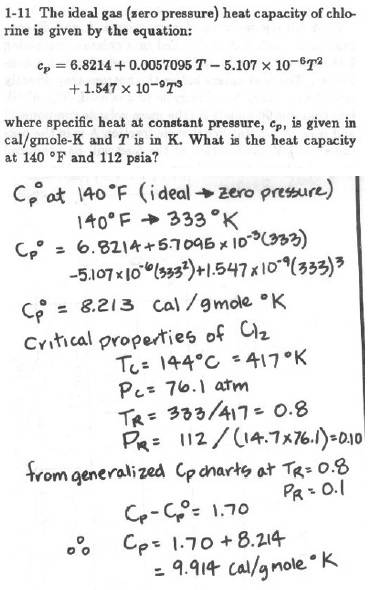At constant temperature, the effect of pressure on molar enthalpy is $$\frac{\partial H}{\partial P}=V-T\frac{\partial V}{\partial T}$$This is used in conjunction with $$PV=zRT$$This leads to $$H(T,P)=H(T,0)-RT^2\int_0^P{\frac{1}{P}\frac{\partial z}{\partial T}dP}$$The heat capacity can be determined by taking the partial derivative of the enthalpy with respect to temperature at constant pressure. The resulting integrals are evaluated using the principle of corresponding states.

At constant temperature, the effect of pressure on molar enthalpy is $$\frac{\partial H}{\partial P}=V-T\frac{\partial V}{\partial T}$$This is used in conjunction with $$PV=zRT$$This leads to $$H(T,P)=H(T,0)-RT^2\int_0^P{\frac{1}{P}\frac{\partial z}{\partial T}dP}$$The heat capacity can be determined by taking the partial derivative of the enthalpy with respect to temperature at constant pressure. The resulting integrals are evaluated using the principle of corresponding states.
that's for calculating the departure enthalpy from ideal, i.e. enthalpy difference for real gas. the question is asking for heat capacity only

that's for calculating the departure enthalpy from ideal, i.e. enthalpy difference for real gas. the question is asking for heat capacity only
What did I say in my last sentence? Do you not know how to take the partial derivative of my enthalpy equation with respect to temperature?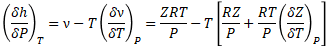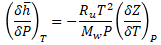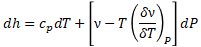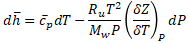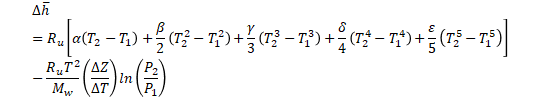I've used these in a previous example from the same book about real gas departure function. This problem in the OP stumped me.

$$C_p=C_p^0-2RT\int_0^P{\frac{1}{P}\frac{\partial z}{\partial T}dP}-RT^2\int_0^P{\frac{1}{P}\frac{\partial^2 z}{\partial T^2}dP}$$

$$C_p=C_p^0-2RT\int_0^P{\frac{1}{P}\frac{\partial z}{\partial T}dP}-RT^2\int_0^P{\frac{1}{P}\frac{\partial^2 z}{\partial T^2}dP}$$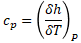View attachment 285805
Are you saying that you agree with the equation I wrote in post #2, but you don't know how I arrived at the equation in post #6?

Starting with the equation in post #2, $$C_p(T,P)=\frac{\partial H(T,P)}{\partial T}$$ and $$C_p^0(T)=\frac{\partial H(T,0)}{\partial T}$$

Starting with the equation in post #2, $$C_p(T,P)=\frac{\partial H(T,P)}{\partial T}$$ and $$C_p^0(T)=\frac{\partial H(T,0)}{\partial T}$$
i figured that out as the functions are H(T,0) for Cp°. how did you get the last term in post#6?

This equation assumes that the partial derivative of z with respect to T at constant P is independent of P (which is incorrect).
i think for that example the pressure remained the same at 25atm and Z was interpolated. the gas (nitrogen) changed from 1atm and 298K to 25atm and 973K, i.e. simultaneous change in T & P. looking to see if departure from ideal was significant at end conditions, not so in this case.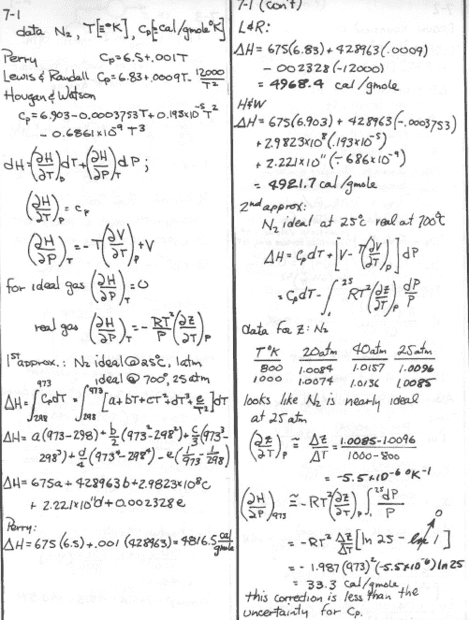i figured that out as the functions are H(T,0) for Cp°. how did you get the last term in post#6?
It's just math. Taking the partial derivative of the overall term with respect to temperature.

It's just math. Taking the partial derivative of the overall term with respect to temperature.
thanks my calculus is very rusty, hence brushing up on my thermo. i think its the chain rule for partial derivatives. as long as I'm satisifed and made everything water tight, I'm happy. thanks again

It's just math. Taking the partial derivative of the overall term with respect to temperature.
i'm stuck on finalising the equation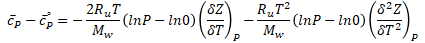how the author arrived at 1.7cal/molK is tricky. do i have to integrate out the partial derivatives? I'm confused, the ln0 term is also spitting out errors

Last edited:
i'm stuck on finalising the equation
View attachment 285834
how the author arrived at 1.7cal/molK is tricky. do i have to integrate out the partial derivatives? I'm confused, the ln0 term is also spitting out errors
Your math is incorrect in several respects. What is the exact word-for-word statement of the problem you are trying to solve?

Your math is incorrect in several respects. What is the exact word-for-word statement of the problem you are trying to solve?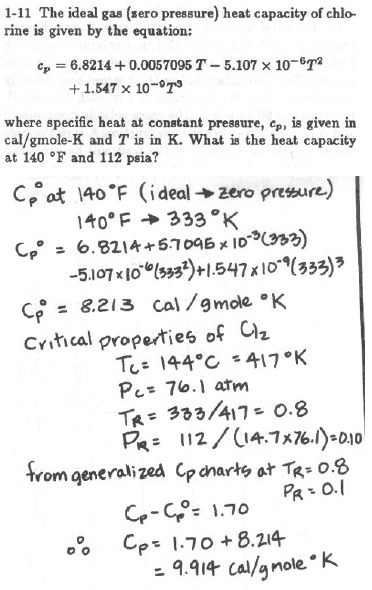hi the top part which isn't hand written

View attachment 285844
hi the top part which isn't hand written
OK. There must be a chart in the book of ##\frac{C_p-C_p^0}{R}## as a function of reduced temperature and reduced pressure. This is what they must have used.

OK. There must be a chart in the book of ##\frac{C_p-C_p^0}{R}## as a function of reduced temperature and reduced pressure. This is what they must have used.
its from the american professional engineers exam set by Randall robinson. the accompanying reference manual doesn't include this chart. the only chart included in the thermo section is the nelson-obert generalized compressibility chart

its from the american professional engineers exam set by Randall robinson. the accompanying reference manual doesn't include this chart. the only chart included in the thermo section is the nelson-obert generalized compressibility chart
Well, you are going to need that chart I mentioned to solve it, or you are going to have to numerically (manually) differentiate the enthalpy departure function at the desired reduced pressure with respect to reduced temperature.

Well, you are going to need that chart I mentioned to solve it, or you are going to have to numerically (manually) differentiate the enthalpy departure function at the desired reduced pressure with respect to reduced temperature.
is there a way to translate this from the chart in post #16?

is there a way to translate this from the chart in post #16?
Something like that, but your charts don't have enough resolution to get what you want. See Fig. 6.6 of Introduction to Chemical Engineering Thermodynamics by Smith and Van Ness.

Something like that, but your charts don't have enough resolution to get what you want. See Fig. 6.6 of Introduction to Chemical Engineering Thermodynamics by Smith and Van Ness.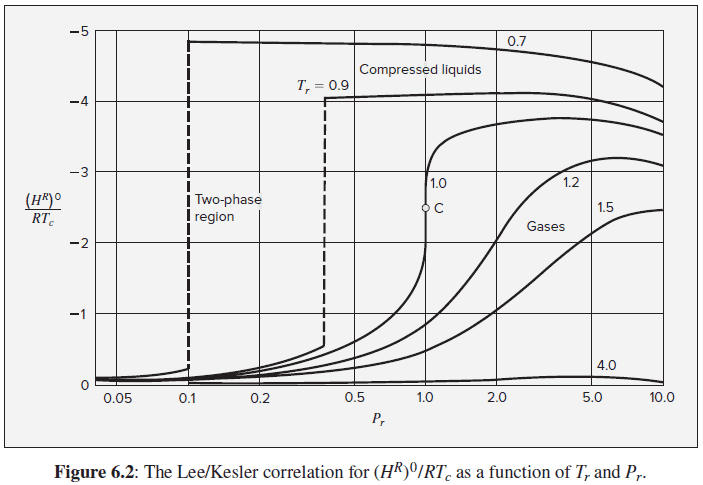i assume its this from the 8th ed. So Tr = 0.8 and Pr = 0.1.. more confused the deeper i go

actually from older 4th ed.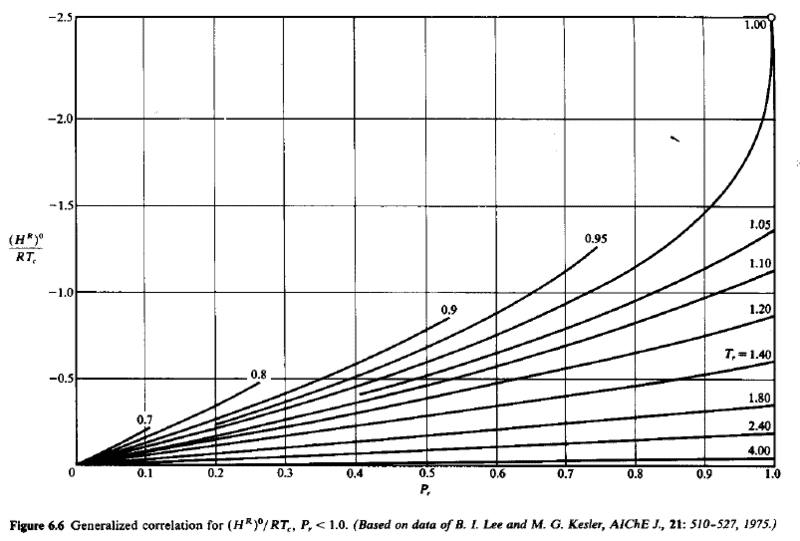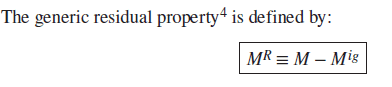say the left hand term is -0.18?

Last edited:
View attachment 285849
i assume its this from the 8th ed. So Tr = 0.8 and Pr = 0.1.. more confused the deeper i go

actually from older 4th ed.
View attachment 285850

View attachment 285851
say the left hand term is -0.18?
The partial derivative of the ordinate with respect to the reduced temperature (evaluated at reduced temperature of 0.8) at constant reduced pressure (abscissa = 0.1) is the residual heat capacity divided by R at the desired conditions. The partial derivative evaluated this way should come out to about +0.85 (dimensionless) if their answer is correct.

Last edited:
The partial derivative of the ordinate with respect to the reduced temperature (evaluated at reduced temperature of 0.8) at constant reduced pressure (abscissa = 0.1) is the residual heat capacity divided by R at the desired conditions. The partial derivative evaluated this way should come out to about +0.85 (dimensionless) if their answer is correct.
so,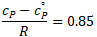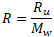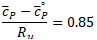Ru = 1.987 cal/molK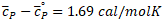=> agrees with solution
ok fine, still need to clarify the jump between the generalized correlation chart to how +0.85 was obtained

If ##y(p_r,T_r)## is the ordinate on the plot, then a 2nd order accurate numerical approximation to the residual heat capacity is $$\frac{C_p^R}{R}=\frac{y(0.1,0.9)-y(0.1,0.7)}{0.9-0.7}$$

From the graph, it looks like the authors overestimated the heat capacity departure (aka residual heat capacity) by a factor of about 2.

From the graph, it looks like the authors overestimated the heat capacity departure (aka residual heat capacity) by a factor of about 2.
it looks like there's another graph with the superscript 1 for the ordinate. i think this takes the acentric factor into account. for chlorine ω=0.073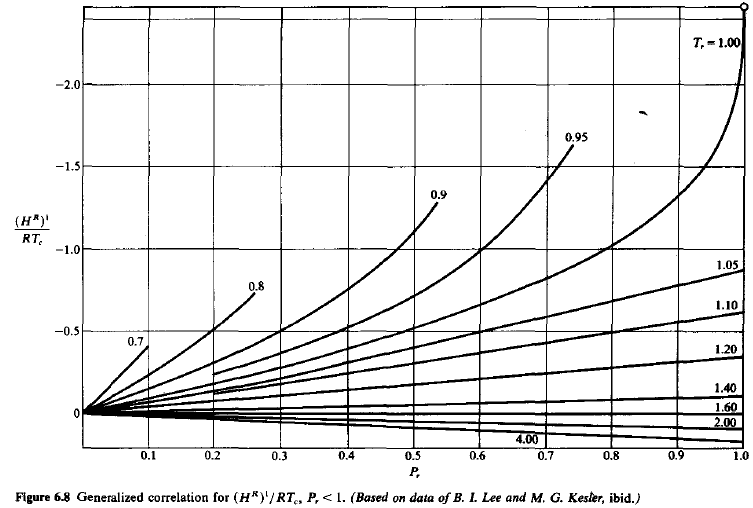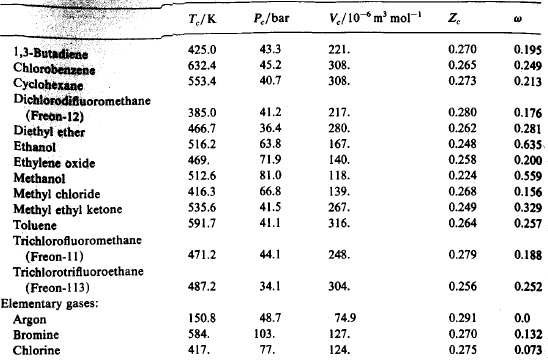Last edited:
•Chestermiller
it looks like there's another graph with the superscript 1 for the ordinate. i think this takes the acentric factor into account. for chlorine ω=0.073
View attachment 285874
View attachment 285875
You're right. It looks like that would substantially reduce the disagreement.

If ##y(p_r,T_r)## is the ordinate on the plot, then a 2nd order accurate numerical approximation to the residual heat capacity is $$\frac{C_p^R}{R}=\frac{y(0.1,0.9)-y(0.1,0.7)}{0.9-0.7}$$
i'm confused, the ordinate is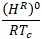with enthalpy, H explicitly stated. how have you arrived at this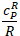? where has the critical temp, Tc term disappeared to?
I've also found this equation in perry's but nothing to convert to cp: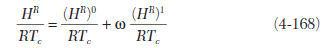i'm confused, the ordinate is View attachment 285928with enthalpy, H explicitly stated. how have you arrived at this View attachment 285929? where has the critical temp, Tc term disappeared to?
I've also found this equation in perry's but nothing to convert to cp:
View attachment 285930
$$\frac{\partial \left(\frac{H^R}{RT_c}\right)}{\partial T_r}=\frac{\partial \left(\frac{H^R}{RT_c}\right)}{\partial (T/T_c)}=\frac{1}{R}\frac{\partial H^R}{\partial T}=\frac{C_p}{R}$$

Regarding Eqn. 4-168, you differentiate each term separately

•gmaverick2k
$$\frac{\partial \left(\frac{H^R}{RT_c}\right)}{\partial T_r}=\frac{\partial \left(\frac{H^R}{RT_c}\right)}{\partial (T/T_c)}=\frac{1}{R}\frac{\partial H^R}{\partial T}=\frac{C_p}{R}$$

Regarding Eqn. 4-168, you differentiate each term separately
ok, using plot digitizer for points on both graphs..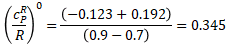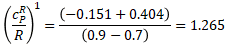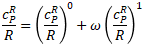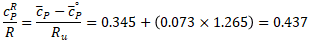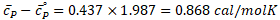Yes, it looks like the author has overestimated the heat capacity by a factor of two assuming this is the correct methodology. I wish authors didn't skip on the working outs. i guess the fun is in the connecting of dots..
Thanks

#### Attachments

•Chestermiller